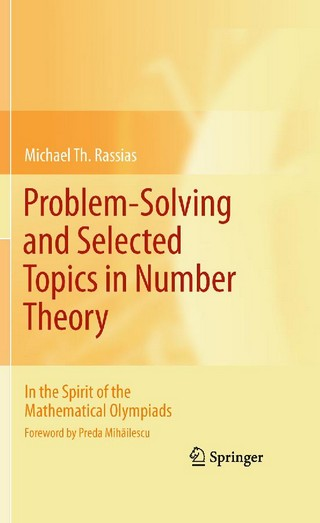Book Toankho

Sách hay - Sách đẹp

Problem-Solving and Selected Topics in Number Theory (In the Spirit of the Mathematical Olympiads)The book provides a self-contained introduction to classical Number Theory. All the proofs of the individual theorems and the solutions of the exercises are being presented step by step. Some historical remarks are also presented. The book will be directed to advanced undergraduate, beginning graduate students as well as to students who prepare for mathematical competitions (ex. Mathematical Olympiads and Putnam Mathematical competition).